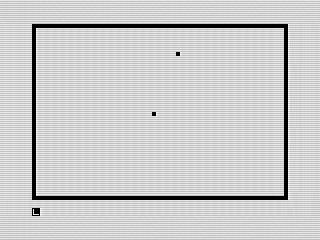# February’s Program: Golf

Play 18 holes of golf in this simple graphic/physics game.This month’s program is a simple game of Golf. Although not overly exciting, it does show off a few ZX81 BASIC features.

One oddity of the ZX81 is that π (pi) is built in, something I haven’t seen in other languages. In this case, it is used to calculate the direction of the golf ball. I also used the `PLOT` and `UNPLOT` commands in this program, something I generally avoided due to speed constraints.

If you take a look at the listing, you’ll notice lines like this:

` 130 LET A1=(A1<1)+(62 AND A1>62)+(A1 AND (A1>=1 AND A1<=62)) 140 LET B1=(B1<1)+(42 AND B1>2)+(B1 AND (B1>=1 AND B1<=42))`

Although ZX81 BASIC didn’t have an `ELSE` or `SWITCH` or the ability to execute multiple lines of code within an `IF` statement, it did however understand boolean logic. You would get 1 if true, and 0 if false. The lines above are using this to validate if the ball is out of bounds and, if so, ensure it stays on the screen. The `A1<1` for instance would return 1 if the ball was less than 1, keeping it on the border. Otherwise, it is 0. This is added to the next check, `(62 AND A1>62)`. Now, in this case, if a constant or variable is passed, it returns that number. That is if `A1>62`, then it returns 62. Neat, huh? The last part returns the value of `A1` if it is inside the bounds. This use of boolean can be used in anywhere a number can be. Although simple in many ways, ZX81 BASIC offered some unique features that more advanced languages lack. Until next month, keep programing!